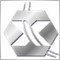# Indicator to EA problem29

Hi everybody,

I'm a beginner with automated trading. I hope to have your help because I'm stuck.
I'm trying to convert this indicator to EA.

This is a breakout strategy based on an interval in hours.

My code does not work, it does not respect the buy signal

ps: sorry for my English, I'm French
```extern string HeureFin = "6:00"; // Fin de la période à analyser
extern int HeureBack = 2; // Durée de la période à analyser
extern double T1 = 250; // Niveau de Take profit
extern double Lot = 0.1;
int MagicNumber = 090855;
double Slippage = 3;
double high, low; // Le + haut et le + bas à analyser
datetime left, right, t99;
int b1, b2;

//+------------------------------------------------------------------+
//| expert initialization function                                   |
//+------------------------------------------------------------------+
int init()
{

return(0);
}
//+------------------------------------------------------------------+
//| expert deinitialization function                                 |
//+------------------------------------------------------------------+
int deinit()
{

return(0);
}
//+------------------------------------------------------------------+
//| expert start function                                            |
//+------------------------------------------------------------------+
int start()
{

// LE TEMPS
right = StrToTime (TimeToStr (TimeCurrent(),TIME_DATE) + " " + HeureFin);

if (right > TimeCurrent())
{
right = StrToTime (TimeToStr (TimeCurrent()- D'1970.01.01 23:59:59',TIME_DATE) + " " + HeureFin);
}
if (TimeDayOfWeek (right)==0)
{
t99   = StrToTime (TimeToStr  (TimeCurrent()- D'1970.01.01 23:59:59',TIME_DATE) + " " + HeureFin )  ;
right = StrToTime (TimeToStr ( t99 + D'1970.01.01 1'));
}

left = StrToTime (TimeToStr ( right - D'1970.01.01 1'* HeureBack ));

if (TimeDayOfWeek (left) == 0)
{
left = StrToTime (TimeToStr ( right - D'1970.01.01 1'* (HeureBack +48) ));
}

b1=iBarShift(NULL, 0, left);
b2=iBarShift(NULL, 0, right);

high = High [iHighest(NULL,0, MODE_HIGH,b1-b2, b2)];
low =Low [Lowest (NULL, 0, MODE_LOW , b1-b2, b2)];
double CloseCandle = iClose(NULL,0,0);

double SigSell= CloseCandle < low;
double SL = high - low;
double Ticket;

{

Ticket = OrderSend(Symbol(), OP_BUY, Lot, Ask, Slippage, low, high + T1*Point, "Buy(#" + MagicNumber + ")", MagicNumber, 0, DodgerBlue);

}

//----
return(0);
}
//+------------------------------------------------------------------+

{
if (OrdersTotal()>=1) // si le nombre total d'ordres ouverts est > 1 alors
{
return(true); // vrai
}
else
{
return(false); // sinon faux
}
}```
Files:6704

You should adjust your TP & SL to work with both 4 digit and 5 digit Brokers.

You should also check the return values from the OrderSend() function calls to make sure it has worked,

`bool isTrade () // VERIFICATION SI UN TRADE EST EN COURS`

Check the trades with the function29

Thank you for your answer, actually the problem is that it does not respect the time to trade. Here starting from 6:00, it does not take into account the highest and lowest29

nobody? =(6704

nobody? =(

patient

```extern double     HourDayStart = 6;

//+------------------------------------------------------------------+
int start()
{
//---- New Day
datetime now = Time,
bod = now - now % 86400,  // Beginning of the day
HourDay = bod + HourDayStart * 3600;   //   6:00

//----
return(0);
}  ```24768

Thank you for your answer, actually the problem is that it does not respect the time to trade. Here starting from 6:00, it does not take into account the highest and lowest

Forgot your code and tell what's the EA should do?29

I'm sorry, but I'm a disaster in programming (no logic lol =(), I'm reading the manual, I will not understand much in fact that is why I turned to you24768

I'm sorry, but I'm a disaster in programming (no logic lol =(), I'm reading the manual, I will not understand much in fact that is why I turned to you

You are not disaster in programming. I'm just asking what do you want to do?29

Sorry I did not understand, English is not my language =)

I want an EA based on breakouts. For example:
I setting a time zone in hours, I mark the highest and the lowest.
I expect a candle closes above for a buy signal, below for a sell signal.

The stop loss is placed on the highest for a sell signal, and vice versa for a buy signal.
Gain: should be equal to the difference between the highest and the lowest, with a trailing stop that is triggered once the target is reached to protect gains.29

Up !22323